# 2021 HSC Chemistry Exam Paper Solutions 🧪⚗️🔬🧑‍🔬

Our Chemistry team have been hard at work coming up with the solutions for your 2021 Chemistry HSC Paper woes. Read on to see how you went!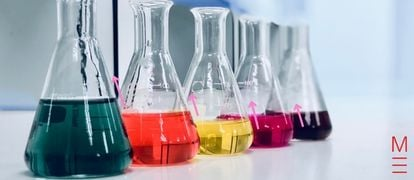Want to find out how you went in the 2021 Chemistry HSC? Well, you’re in the right place. Our Chemistry geniuses have been busy cooking up our 2021 HSC Chemistry Exam Paper Solutions 🧪⚗️🔬🧑‍🔬! Read on to find out how you went!

## 2021 HSC Chemistry Exam Paper Worked Solutions

These are the responses to the 2021 Chemistry paper, which can be found here on the NESA website.

## Section 1: Multiple Choice Questions

1 A At dynamic equilibrium, the rate of the forward and reverse reaction are equal.
2 B Silver nitrate produces the ions Ag+ and NO3. Based on the solubility rules, all nitrates are soluble (NO3), so the precipitation reaction will involve Ag+. Of the available ions, Agwill form a precipitate with Cl.
3 A The compound shown is a secondary amide. The prefix for the compound name is given by the longest carbon chain (propan-) and the suffix is given by the functional group (-amide). The alkyl chain that is bound to the amide nitrogen is treated as a substituent, and is named by using an uppercase, italicised N to indicate that the substituent is attached to the nitrogen atom (N-methyl). As a result, the name of the compound is N-methylpropanamide.
4 C Esterification involves the reaction of a carboxylic acid and an alcohol to produce an ester. The ester is named using the scaffold alkyl alkanoate, where the alkyl component of the compound originates from the alcohol, and the alkanoate component originates from the carboxylic acid. As a result, ethyl pentanoate is synthesised through the reaction of ethanol and pentanoic acid.
5 B The method used by the student contains two errors:

a. The student rinses the burette with deionised water rather than the titrant (sodium hydroxide). This would result in the titrant solution being diluted, meaning that a larger titre would be required to reach the end point. As a result, the measured concentration of acetic acid would appear higher than the actual concentration.
b. The student rinses the conical flask with the unknown solution (acetic acid). This would result in a greater number of moles of the unknown solution being present in the conical flask, requiring a larger titre to reach the end point. As a result, the measured concentration of acetic acid would appear higher than the actual concentration.

Overall, the calculated concentration of acetic acid would be higher than the actual concentration.

6 C  Ka is only affected by temperature, so will remain the same after dilution. The extent of ionisation increases as the solution is diluted. The increase in the extent of ionisation can be understood by first considering the equilibrium for the ionisation of a weak acid and the equilibrium constant expression:
HA(aq) ⇋ H+(aq) + A(aq)    Ksp = [H+][A]/[HA]
By diluting the solution, the concentrations of [H+], [A], and [HA] will all decrease. As a result, the reaction quotient (Q) based on these concentrations will be less than the equilibrium constant (K) (Q < K). Thus, the equilibrium will shift right to produce more ions and increase the extent of ionisation.
7 A Bromomethane will have a lower boiling point than methanol. Methanol has hydrogen bonding as its dominant intermolecular force, while bromomethane has dipole-dipole forces as the dominant intermolecular force. As dipole-dipole forces are weaker than hydrogen bonds, less thermal energy is required to overcome these forces for the substance to boil.
8 D Soap molecules have two components: a hydrophilic head group and a hydrophobic tail. The hydrophobic tail forms stronger adhesive forces with the oil, while the hydrophilic head group forms stronger adhesive forces with water. As a result, the soap molecules orientate so that the tail is contained within the oil and the head group is at the interface of the oil and the water.
9 D The wavelength used in the measurement of an analyte by UV-Vis spectroscopy corresponds to the absorption maximum of the analyte. Consequently, the solvent used to prepare the sample should not have a strong absorbance in this region otherwise it would impact on the measured concentration of the analyte.
10 B The polymer chain shown is a type of condensation polymer known as a polyester. These polymers are formed through the reaction of monomers containing both a carboxylic acid and a hydroxyl group, resulting in the elimination of water (H2O). Based on the connectivity shown in the polymer chain, the hydroxyl group would need to be located on the carbon adjacent to the carboxylic acid group.
11 C The equilibrium constant expression for this reaction is:

K = [Cl2]

As this expression shows, the equilibrium is independent of the amount of PCl5(s), and only depends on the concentration of Cl2(g). As a result, the addition of PCl5(s) will not disturb the equilibrium.

12 A The m/z peak at 98 in the mass spectrum corresponds to the molecular ion, and  is consistent with a molecular formula of C6H10O. The carbon-13 NMR spectrum depicts four unique 13C environments, indicating that symmetry exists in the compound.
13 C Alkenes under hydration conditions react to form alcohols. The oxidation of a secondary alcohol results in the formation of a ketone.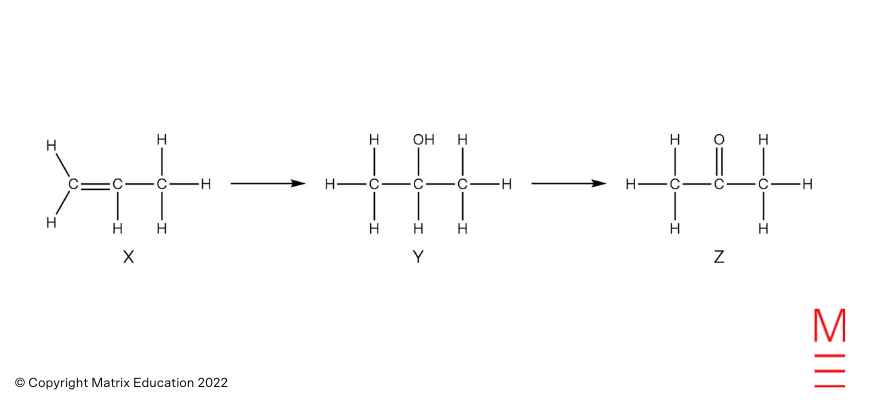14 D  The concentration of the diluted nickel sample is found using the calibration curve, and is approximately 0.0021 mol L-1. The concentration of the undiluted sample is found by:

c(undiluted) = c(diluted) x V(diluted) / V(undiluted)

= (0.0021 x 250) / 10 = 0.0529 mol L-1

n(Ni) = c x V

= 0.0529 x 0.050 = 0.002645 mol

m(Ni) = n x MM

= 0.002645 x 58.69 = 0.16 g which is closest to 0.15 g.

15 B HCl(aq) + NaOH(aq) NaCl(aq) + H2O(aq)

n(HCl) = c x V = 0.20 x 0.020 = 0.0040 mol

n(NaOH) = c x V = 0.50 x 0.020 = 0.010 mol

Therefore, HCl is limiting.

n(NaOH) remaining = 0.010 – 0.0040 = 0.0060 mol

c(NaOH) = n / V = 0.0060 / 0.040 = 0.15 mol L-1 = [OH]

pOH = -log10[OH] = -log10(0.15) = 0.8239

pH = 14 – pOH = 14 – 0.8239 = 13.2

16 B

The titration curve depicts equivalence points at approximately 10.0 mL and 20.0 mL. Since there are two equivalence points, the acid is likely diprotic.

17 D Based on the mole ratio of phosphate in each compound:

n(Na3PO4) = n((NH4)3PO412MoO3)

n((NH4)3PO412MoO3) = m / MM = 24.21 / 1877 = 0.012898 mol = n(Na3PO4)

m(Na3PO4) = n x MM = 0.012898 x (3 x 22.99 + 30.97 + 4 x 16.00) = 2.115 g

18 D

 Label Multiplicity Number of hydrogens a Singlet 3 b Quartet 4 c Triplet 3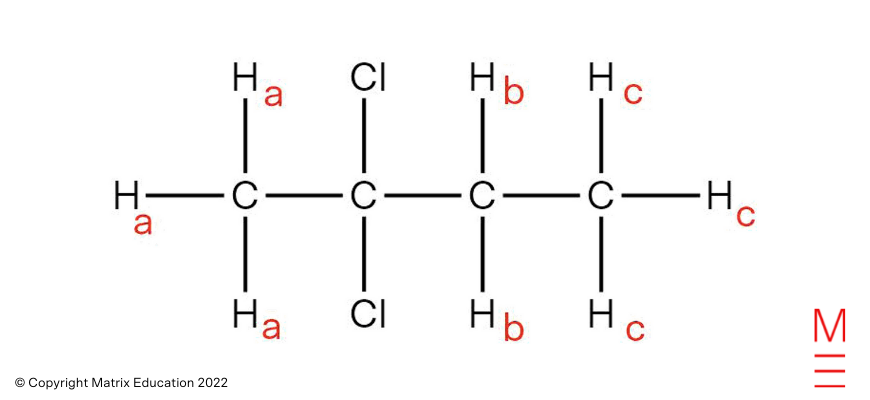19 C  2AgNO3(aq) + K2SO4(aq) Ag2SO4(s) + 2KNO3(aq)

Ag2SO4(s) ⇋ 2Ag+(aq) + SO42-(aq)

Ksp = [Ag+]2[SO42-] = 1.20 x 10-5   → [Ag+] = 1.20 10-50.100 = 0.01095 mol L-1

n(Ag+) = c x V = 0.01095 x 0.250 = 0.002739 mol = n(AgNO3)

m(AgNO3) = n x MM = 0.002739 x 169.9 = 0.465 g

20 C  [(CH3)3NH]+(aq) + H2O(l) ⇋ H3O+(aq)  + (CH3)3N(aq)

Ka = [H3O+][(CH3)3N] / [[(CH3)3NH]+] = 1.55 x 10-10

pH = 4.46 → [H3O+] = 10-4.46 = [(CH3)3N] (based on stoichiometry)

[[(CH3)3NH]+] = [H3O+][(CH3)3N] / Ka = [10-4.46][10-4.46] / 1.55 x 10-10 = 7.7565 mol L-1

In a saturated solution of trimethylammonium chloride:

[(CH3)3NH]Cl(s) ⇋ [(CH3)3NH]+(aq) + Cl(aq)

Ksp = [[(CH3)3NH]+][Cl]

[Cl] = [[(CH3)3NH]+] = 7.7565 mol L-1 (based on stoichiometry)

Therefore, Ksp = (7.7565)(7.7565) = 60.2

## Want to learn how to produce solutions like these?

Matrix Chemistry courses have in-depth resources and structured theory lessons taught by experts to ensure you are HSC ready!

## Section 2: Long Response Questions

### Question 21(a)

Safety concern: Organic liquids are volatile and flammable, meaning they can catch on fire and sustain combustion. To address: ensure there are no open flames and keep away from ignitable sources. Work in fume hood.

### Question 21(c)

Hex-1-ene: bromine test. Immediate decolourisation of bromine from red-brown to colourless would be observed.

### Question 22

Addition of heat disturbs the equilibrium. According to Le Chatelier’s principle, the equilibrium shifts left in the endothermic direction to remove added heat and minimise the disturbance.

Addition of NaOH, results in a decrease in concentration of H+ as OH(aq) + H+(aq) H2O(l). This disturbs the equilibrium. According to Le Chatelier’s principle, the equilibrium shifts left in to replace H+ and minimise the disturbance.

### Question 23(a)

HCOOH(aq) + KOH(aq) KHCOO(aq) + H2O(l)

### Question 23(b)

Basic.

Dissolving: KHCOO(s)    K+(aq)  +  HCOO(aq)

Reacting with water: HCOO(aq)+  H2O(l)  ⇌  HCOOH(aq)  +  OH(aq)

KHCOO dissolves and produces HCOO(aq) which is basic as it is the conjugate base of a weak acid. It reacts with water to produce OH(aq), increasing the pH and forming a basic solution.

### Question 24

 Structural formula Name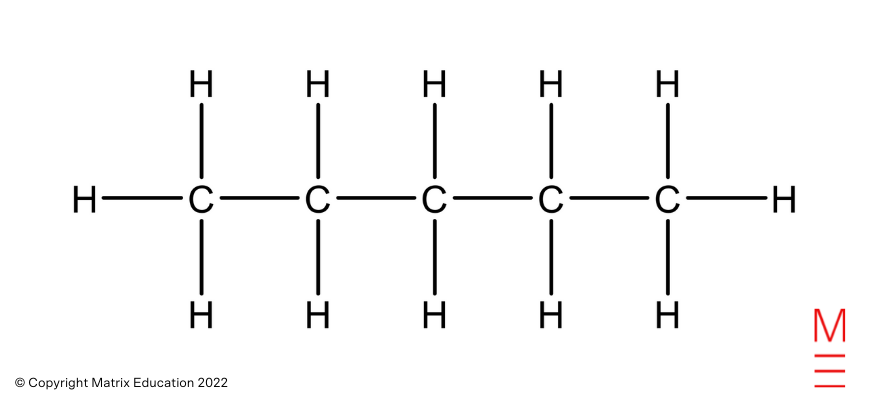Pentane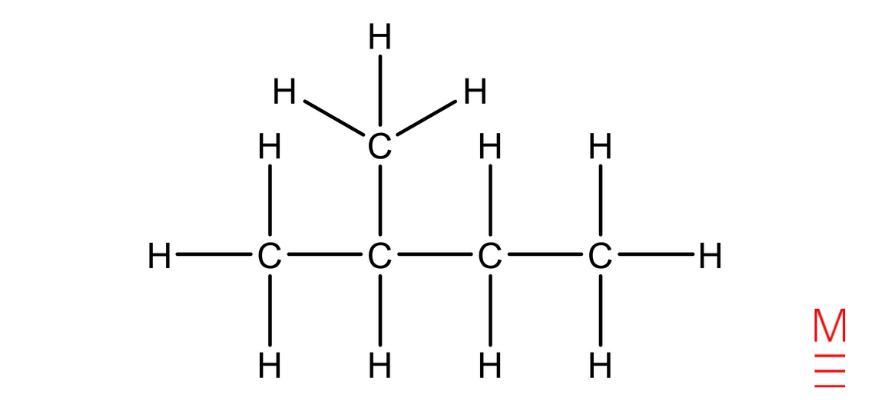2-methylbutane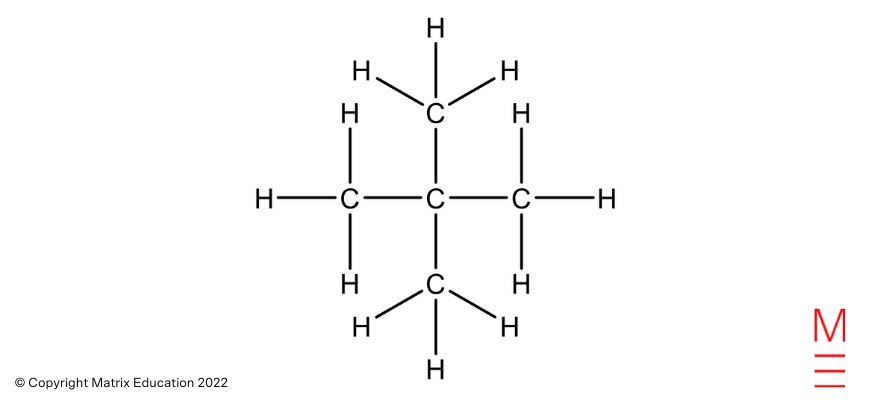2,2-dimethylpropane

### Question 25

C6H12O6(aq)         2C2H5OH(aq)     +     2CO2(g)

V(CO2) = 1006 mL = 1.006 L

n(CO2) = V/MV = 1.006 / 24.79 = 0.040580 mol

n(C2H5OH) = n(CO2) = 0.040580 mol

m(C2H5OH) = n x MM = 0.020855 mol x (12.01 x 2 + 1.008 x 6 + 16.00) g mol-1 = 1.87 g (3 s.f)

### Question 26(a)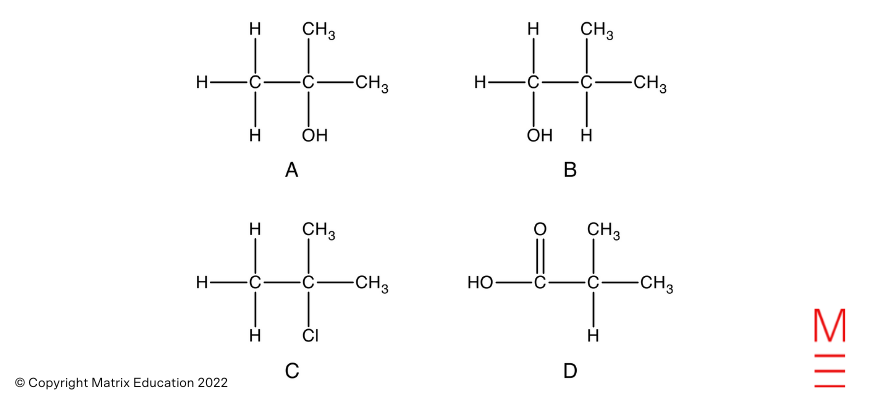### Question 26(b)

Esterification is slow at room temperature, hence heating is required to increase the rate. The reactants and products are also volatile. Hence reflux is used to allow for gentle heating without the loss of reactants and products.

### Question 27(a)

Ksp = [Li+]3[PO43-]

Precipitation occurs between sample 3 and 4.

Sample 3:

Lower Ksp = 0.153 x 0.01 = 3.4 x 10-5 (2 s.f.)

Sample 4:

Higher Ksp = 0.153 x 0.10 = 3.4 x 10-4 (2 s.f.)

Range = 3.4 x 10-5 to 3.4 x 10-4

### Question 27(b)

More increments in the concentration of phosphate could be used which would allow the range to be narrowed.

Alternatively, turn into precipitation titration.

### Question 28

2MOH(aq)   +   Cu(NO3)2(aq)     Cu(OH)2(s)   +   2MNO3(aq)

n(Cu(OH)2) = m / MM = 4.61 g / (63.55 + 16.00 x 2 + 1.008 x 2) g mol-1 = 0.04725 mol

n(MOH) = 2 x n(Cu(OH)2) = 0.0945 mol

MM(MOH) = m(MOH) / n(MOH) = 5.30 g / 0.0945 mol = 56.084577 g mol-1

MM(M) = MM(MOH) – MM(OH) = 56.084577 – (16.00 + 1.008) = 39.1 g mol-1 (3 s.f.)

Alkali metal hydroxide = Potassium hydroxide, KOH

### Question 29

IR Spectrum: The strong absorption between 3000 – 3700 cm-1 is characteristic of N-H bonds in amines. This is present in pentane-1,5-diamine.

Mass Spectrum: The fragment ion with a m/z ratio of 30 corresponds to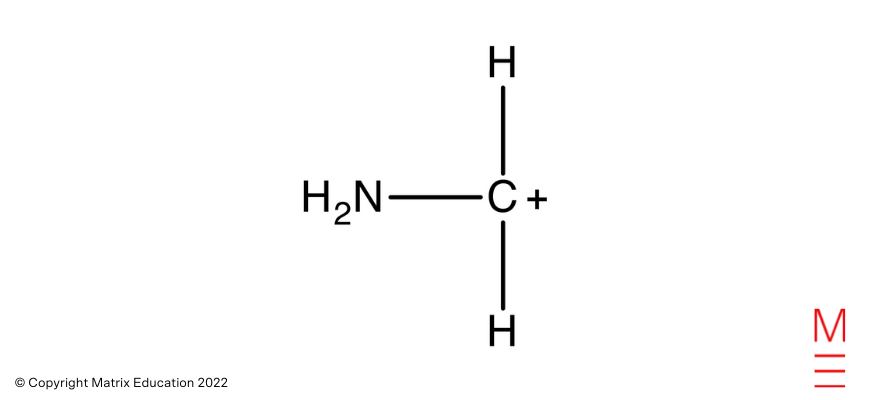Which is obtained from the fragmentation of pentane-1,5-diamine as shown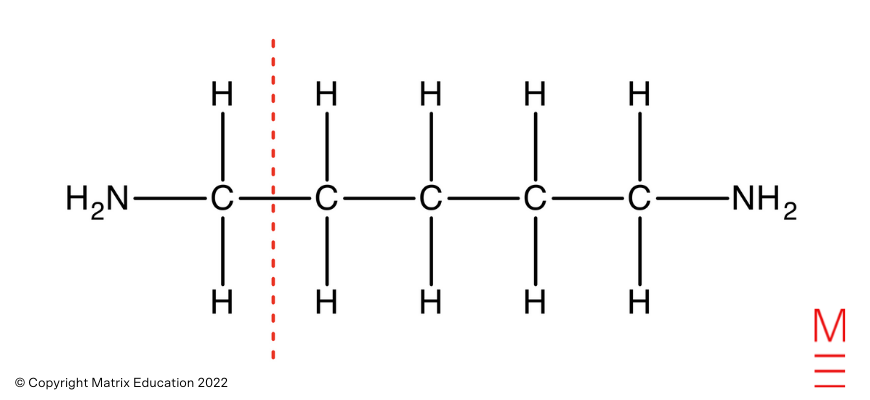Carbon-13 NMR Spectrum: There are three peaks in the 13C NMR spectrum, representing 3 unique carbon environments. This is consistent with pentane-1,5-diamine as shown: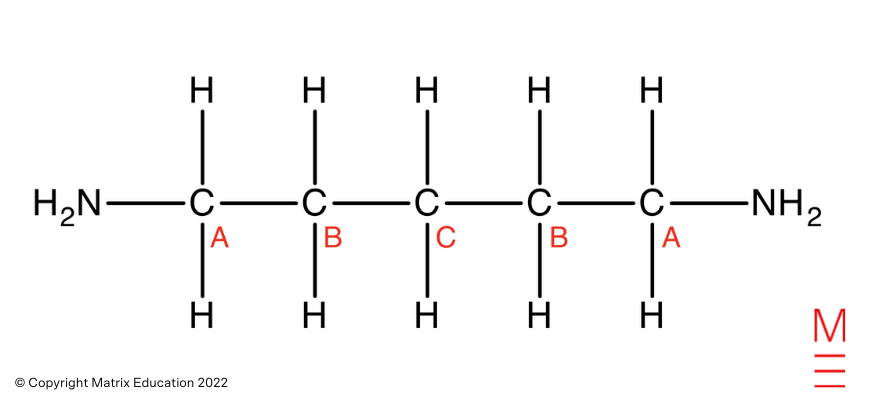“A” carbons correspond to the most downfield peak, which falls within the characteristic chemical shift range for carbons directly bonded to amines. The “A” carbons are directly bonded to an electronegative nitrogen which withdraws electron density from the carbons. This causes these carbons to be deshielded and experience more of the external magnetic field, hence absorbs a higher frequency of radiation.

“B” carbon corresponds to the middle peak, which falls within the characteristic chemical shift range for aliphatic carbons. Carbon B is the one carbon away from the amine, hence will be more shielded than the A carbons. Therefore, the B carbons experience less of the external magnetic field and absorb a lower frequency of radiation.

“C” carbon corresponds to the most upfield peak, which falls within the characteristic chemical shift range for aliphatic carbons. Carbon C is the furthest away from the amine, hence will be most shielded and therefore will experience less of the external magnetic field. Consequently, Carbon C absorbs a lower frequency of radiation.

Proton NMR Spectrum: The overlapping quintets correspond to the hydrogens on B carbons and C carbon. The quintet splitting indicates that the hydrogens neighbour 4 chemically equivalent protons in a different chemical environment.

### Question 30

The addition of aqueous sodium chloride is not useful for identifying any of the ions. Na+ ion does not precipitate with OH or CH3COO ions. Similarly, Cl ion does not precipitate out Ba2+, Ca2+ or Mg2+ ions.

The addition of aqueous silver nitrate is useful for distinguishing between OH and CH3COO ions. Silver ions react with OH ions to produce a brown precipitate, which dissolves in dilute HCl. Although acetate ions can precipitate with silver ions, the precipitate is less soluble and is white, thus can be easily distinguished.

The addition of aqueous sodium sulfate is not a useful test to be used alone. The sulfate ion can precipitate out both Ba2+ and Ca2+ ions, and both precipitates are white and cannot be distinguished visually. An additional test, like a flame test, is required to distinguish between Ba2+ and Ca2+ ions. The test allows for Mg2+ ions to be eliminated, but cannot definitively identify its presence.

### Question 31

 Concentration (M) N2(g) 3H2(g) 2NH3(g) Initial 0.45 + x 0.1 0.58 Change – 0.0025 – 0.0075 + 0.005 Equilibrium 0.4475 + x 0.0925 0.585


\begin{align*}
K = \frac{[NH_3]^2 }{[N_2][H_2]^3 } &= 748 \\
\frac{0.5852}{((0.4475 + x) \times 0.09253} &= 748 \\
\frac{0.5852}{3.5417} \times 10-4 + 0.09253x &= 748 \\
0.5852 &= 748 (3.5417 \times 10-4 + 0.09253x) \\
0.5852 &= 0.264923 + 0.592006x \\
x = 0.5852 – \frac{0.264923}{0.592006} &= 0.130576 \text{mol /L} \\
\end{align*}

To 10 L, add 1.3 mol (2 s.f.)

### Question 32

In both reactions 1 and 2, a strong acid and strong base are reacted together. A strong acid completely ionises in water to give H+ ions and a strong base completely dissociates to give OH ions. Thus the enthalpy change only reflects the reaction between H+ and OH to produce water. OH(aq) + H+(aq) H2O(l)

Since the net reaction is the same, ΔH is the same.

In reaction 3, a weak acid is reacted with a strong base. A weak acid is not fully ionised in water, hence some intact acid will be present. During neutralisation, energy from the reaction will be absorbed to break the covalent bond in the intact acid to form H+ ions. The enthalpy change therefore reflects both the exothermic reaction between H+ and OH and the endothermic bond breaking process. Hence overall ΔH will be less exothermic.

### Question 33(a)

ΔG = ΔH – TΔS = -95 – (-80) = -15 kJ/mol

### Question 33(b)

At T1, the ΔH < TΔS. Since ΔG = ΔH – TΔS, ΔG < 0. Hence the reaction is spontaneous in the forward direction and non-spontaneous in the reverse direction at this temperature.

At T3, the ΔH > TΔS. Since ΔG = ΔH – TΔS, ΔG > 0. Hence the reaction is non-spontaneous in the forward direction and spontaneous in the reverse direction at this temperature.

At T2, the ΔH = TΔS. Since ΔG = ΔH – TΔS, ΔG = 0. Hence the system is at equilibrium at this temperature.

### Question 34

The pH of water is initially at 7 as it is neutral. As gaseous HCl is bubbled into the water, it dissolves and ionises to give H3O+ ions.

HCl(aq)  + H2O(l)   H3O+(aq)  +  Cl(aq)

As more HCl bubbles into the water, the concentration of H3O+ increases. Since pH = -log10[H3O+], the pH of the solution decreases with increasing concentration of H3O+. The change in the pH between t0 and t1 is steep as the proportional change in the concentration of H3O+ ions is initially large. The change in pH between t1 and t2 is less steep as the proportional change in the concentration of H3O+ ions is less as more acid is added.

The pH of X and Y can be observed to gradually decrease between t0 and t1. This indicates X and Y are buffer solutions, containing a weak acid/base and its conjugate. Since the pH of these solutions at t0 is around pH 5, they are most likely to be composed of a weak acid and the conjugate base.

HA(aq)  +  H2O(l)  ⇌  H3O+(aq)  + A(aq)

When HCl is added and the concentration of H3O+ increases, these solutions are able to resist changes in the concentration of H3O+ as the equilibrium will shift left to remove the added H3O+ to minimise the disturbance, in accordance with Le Chatelier’s principle. Since concentration of H3O+ is relatively unchanged, pH is also relatively unchanged.

In between t1 and t2, the pH decreases more rapidly for both X and Y, indicating the solutions have reached their buffering capacity. Solution X is depleted sooner than Y suggesting X has a lower buffering capacity compared to Y. This may be due to X having a lower concentration of weak acid and its conjugate base, compared to Y.

### Question 35

V(Na2S2O3) = (28.7 + 28.4 + 28.6) / 3 = 28.56667 mL = 0.02856667 L

n(Na2S2O3) = c x V = 0.900 x 0.02856667 = 0.02571 mol = n(S2O32-)

n(I2) = ½ x n(S2O32-) = 0.012855 mol

n(Cr2O72-)excess = ⅓ x n(I2) = 0.004285 mol

n(Cr2O72-)total = c x V = 0.500 x 0.020 = 0.010 mol

n(Cr2O72-)reacted = 0.010 – 0.004285 = 0.005715 mol

n(C2H5OH) = 3/2 x n(Cr2O72-)reacted = 8.5725 x 10-3 mol

c(C2H5OH)diluted = 8.5725 x 10-3 / 0.025 = 0.3429 mol L-1

c(C2H5OH)concentrated = (0.3429 x 1.00) / 0.025 = 13.716 mol L-1

In 1 L:

n(C2H5OH) = 13.716 mol

m(C2H5OH) = n x MM = 13.716 x (12.01 x 2 + 1.008 x 6 + 16.00) = 631.868 g

V(C2H5OH) = 631.868 / 0.789 = 800.8475 mL

% v/v = (800.8475 / 1000) x 100 = 80.1 % v/v (3 s.f.)

Less than 85%, thus does not meet manufacturer’s requirements.

### Question 36

Reaction 1: H2SO3(aq)+  H2O(l)  ⇌  H3O+(aq)  +  HSO3(aq)

Ka1 = [H3O+][HSO3] / [H2SO3] = 10-1.82

Reaction 2: HSO3(aq)+  H2O(l)  ⇌  H3O+(aq)  +  SO32-(aq)

Ka2 = [H3O+][SO32-] / [HSO3] = 10-7.17

Reaction 3: H2SO3(aq)+  2H2O(l)  ⇌  2H3O+(aq)  +  SO32-(aq)

Ka3 = [H3O+]2[SO32-] / [H2SO3]

Ka1 x Ka2 = [H3O+][HSO3] / [H2SO3]  x  [H3O+][SO32-] / [HSO3] = [H3O+]2[SO32-] / [H2SO3]

Therefore,

Ka3 = Ka1 x Ka2 = 10-1.82 x 10-7.17 = 1.0 x 10-9 (2 s.f.)